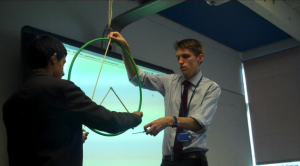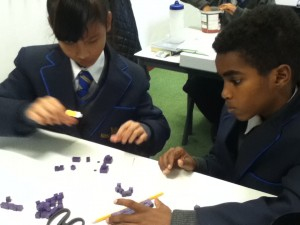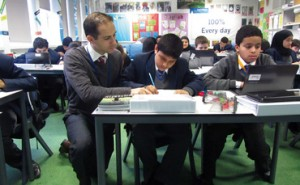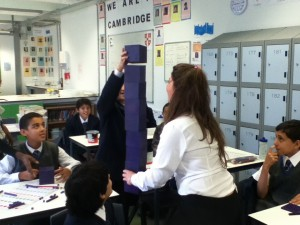# Modelling in mathsI’ve come to the conclusion that ace teachers are experts in classroom culture and that the most effective maths teachers are also excellent at modelling and questioning.

With the hope of getting some feedback in the comments, here’s what I try to do consistently with modelling.

• I start with “empty hands and look this way”. I wait until hands are empty and eyes are looking this way. Every time. I don’t begin modelling until that’s done. I stay on top of low level distraction throughout. From lesson one.
• Where it makes sense I use physical resources to represent the concept. If I’ve got enough sets I try to put these resources in front of every pair of pupils and do some whole-class, quick-fire show-me stuff.• Show me 98 (on an abacus, for example), add on 1 more, 1 more.
• Show me division using place value blocks.
• Show me a parallelogram (using lollipop sticks). Now change it to a rectangle (I’m aware of the overlap between parallelogram and rectangle but that’s usually for another lesson). Show me a square.
• Show me a clockwise rotation (with a ruler or pencil)
• Show me negative 5 (on an air number line)
• If it’s a procedure that has a step in it that I-know-they-can-do-in-isolation-but-they-might-get-stuck-on-because-it’s-now-in-a-new-context, we’ll practice that step as a warm up.For example…

• Simplifying fractions requires finding a common factor. So we’ll warm up by finding common factors.
• Multiplying a pair of brackets requires ability to multiply a variable by a number. So before tackling pairs of brackets we’ll just do y × 3, y × y, -2 × y, etc.
• Working out a scale factor requires some mental or written division. So we’ll practice working out how many times bigger one number is than another.
• If it’s a procedure that has some unfamiliar steps that I know will hold them up, we’ll take out those out and work on them in isolation.
• Determining opp, adj and hyp on a right angle triangle
• Choosing whether to add or subtract equations when solving simultaneous equations by elimination
• Working out frequency density for a histogram
• Working out b² – 4ac for the quadratic formula
• Deciding whether the line should be solid or dashed for graphing inequalities
•• After understanding and becoming successful at each step, we’ll then glue the steps back together. For example, if they’ve got the hang of step 1 and step 2 in isolation of each other, then we’ll do step 1 followed by step 2.
• When choosing examples to demonstrate I think about the following:
• Will the sequence of examples lead them to a false generalisation? For example, if I choose a series of fractions to simplify that are all made up of even numbers, will they assume that simplifying only involves dividing by 2?
• How can I make example 2 very similar but slightly different to example 1 so that they can see the impact of changing one thing? Maybe I’ll change one of the signs, one of the numbers, one of the variables, one of the powers, the order, the orientation of the shape, involve special cases using 0 or 1, introduce a fraction.
• Are them some non-examples that will help to make the point. If I’m trying to exemplify what a polygon, a difference of two squares, an improper fraction, a Pythagorean triple…what counter examples would help my pupils understand the required features of a true example?
• As the series of examples goes on, I’ll tend to leave gaps in the steps or what I’m writing to get their help filling it in.
• When checking how well they’re following the maths, I don’t ask “Does everyone understand?”, I target questions at pupils and lure the less confident into giving me an answer to a gap fill or another easy question.

Or…

• I make the most of whole class responses (“Class, after 3, what is the highest common factor of 12 and 16? 1…2…3…!”) or showing me answers on their fingers or on a mini whiteboard.
• I use 10 second bursts of tell-your-partner
• I use true or false questions, is this right/wrong questions, the awesome question stems from Thinkers (by John Mason and Anne Watson).
•• If there’s another adult in the room, I like to get some dialogue with them in front of the pupils. For example, I might get them to explain the steps on the board while I pretend to be a student with lots of questions. If it’s a TA I regularly work with then I insist they chip in with a question when they think I’ve missed something in my explanation.

## 4 Replies to “Modelling in maths”

1.danpearcymaths.wordpress.com says:

I like this post for lessons appropriate for direct instruction – great AfL techniques throughout. In fact, we also do this in a similar way when getting Grade 6 students used to problem solving/investigation – we teach individual skills (e.g drawing diagrams for word problems, simplifying a difficult problem to begin, organising data in a table, verifying a formula with examples, etc.) in individual lessons, then put them all together for their first investigation in Year 7.

2.Matt Dunbar says:

“How can I make example 2 very similar but slightly different to example 1 so that they can see the impact of changing one thing? Maybe I’ll change one of the signs, one of the numbers, one of the variables, one of the powers, the order, the orientation of the shape, involve special cases using 0 or 1, introduce a fraction.” – Bruno nails the essence of variation theory and by going further … to address special cases using 0 and ±1 … destroys prior and eliminates potential of acquiring new misconceptions.

3.Sharon Malley says:

Last term one of the colleagues showed me the Frayer model for defining concepts it has really made me use non-examples in my teaching – well worth a look.

4.Maria Holmes says:

Modeling for maths is good concept I think that it will make maths learning easy and as maths teacher you will have option to teach…!!!!